Our World Statistics Day conversations have been a great reminder of how much statistics can inform our lives. Do you have an example of how statistics has made a difference in your life? Share your story with the Community!
Choose Language Hide Translation Bar
JMP add-in measures distance between 2 points

JMP has many tools and features that allow you to interactively explore and analyze data. But what if you just want to measure the distance between two points? You could compute the distance with the standard distance formula, but what if the coordinates are latitude and longitude pairs? The distance formula would not be a lot of help then. Thanks to the extensibility of JMP, I was able to develop a new add-in to do one simple task: measure distance. The Distance Tool Add-In is an interactive tool that enables you to perform quick and effortless measurements.

In addition to Euclidean distance, the Distance Tool has various distance metrics for you to select from. The tool can compute:

• Euclidean distance

• Absolute difference between coordinate components

• Taxicab distance

• Great-circle distance

• Other various distance metrics

The tool first finds all graphs that contain objects with measurable distances (Figure 1). The graphs are then assigned a unique key value based on the window title and position. You can then make measurements in the current graph by simply clicking and dragging (Figure 2).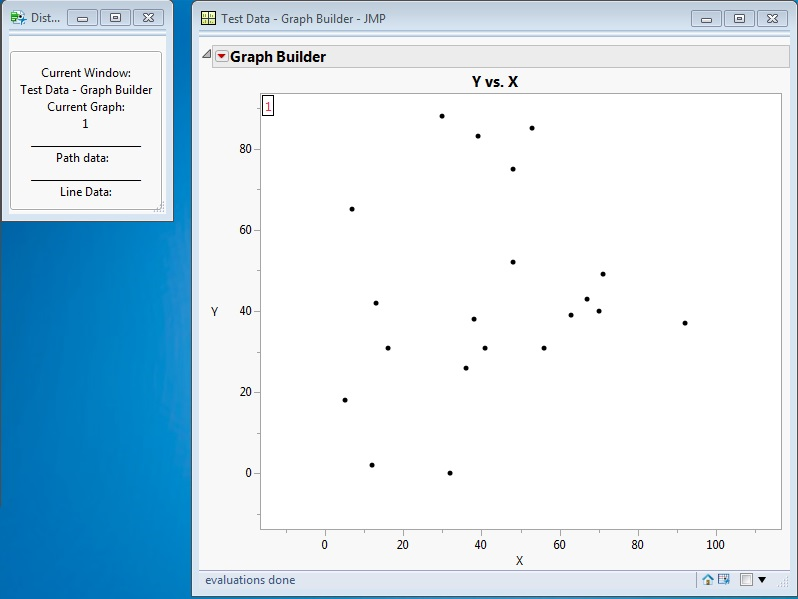Figure 1: The original graph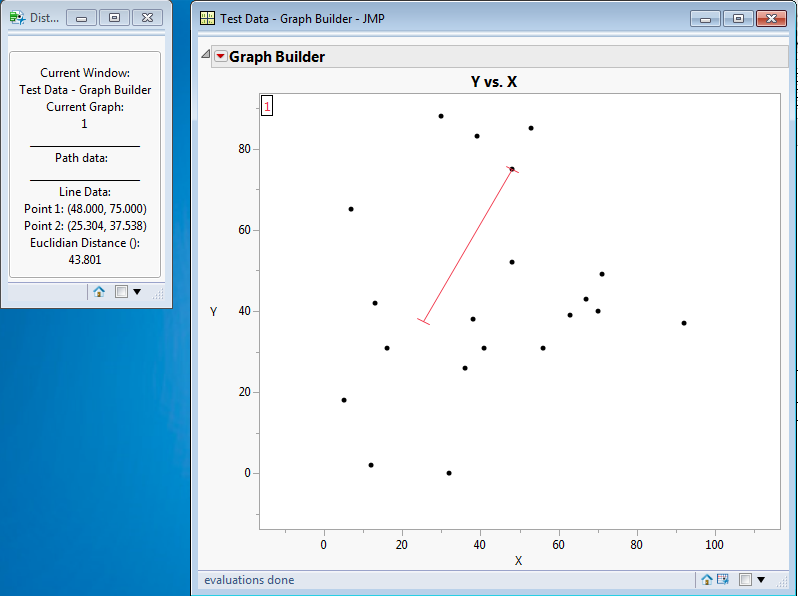Figure 2: Euclidian measure between two points

Now what if you have a graph with latitude and longitude as its axes? No problem. The Great-Circle metric allows you to measure geographic distances between geodesic coordinates (Figure 3).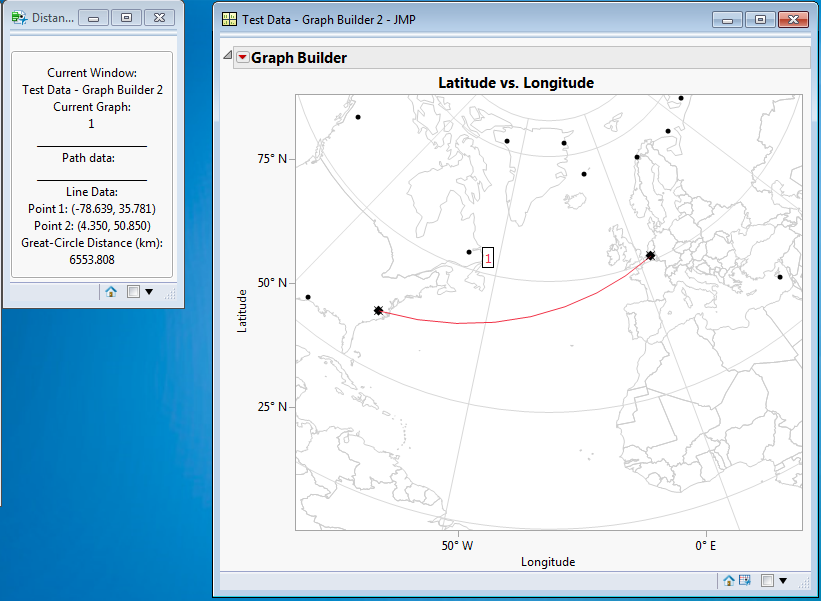Figure 3

With the tool’s custom scale feature, you can even set your own scale for graphs with arbitrary axes.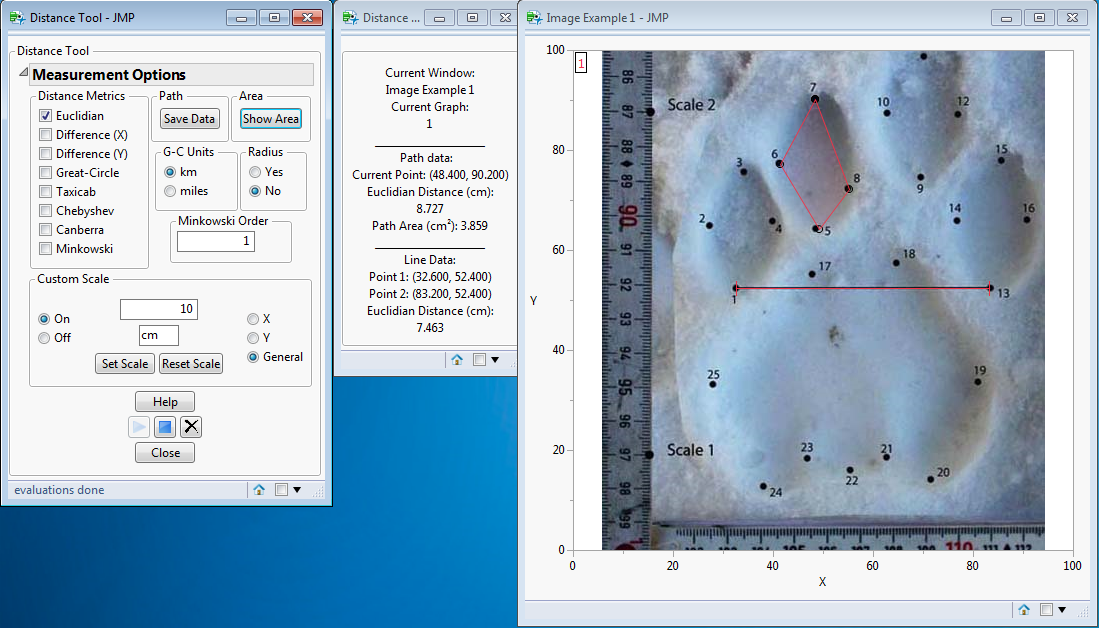Figure 4

The tool even allows you to trace out a path or polygon shapes, as in the image of an animal footprint (Figure 4).

All the measurements are recorded in separate data tables to give you the ability to store, analyze and organize the information you want (Figure 5).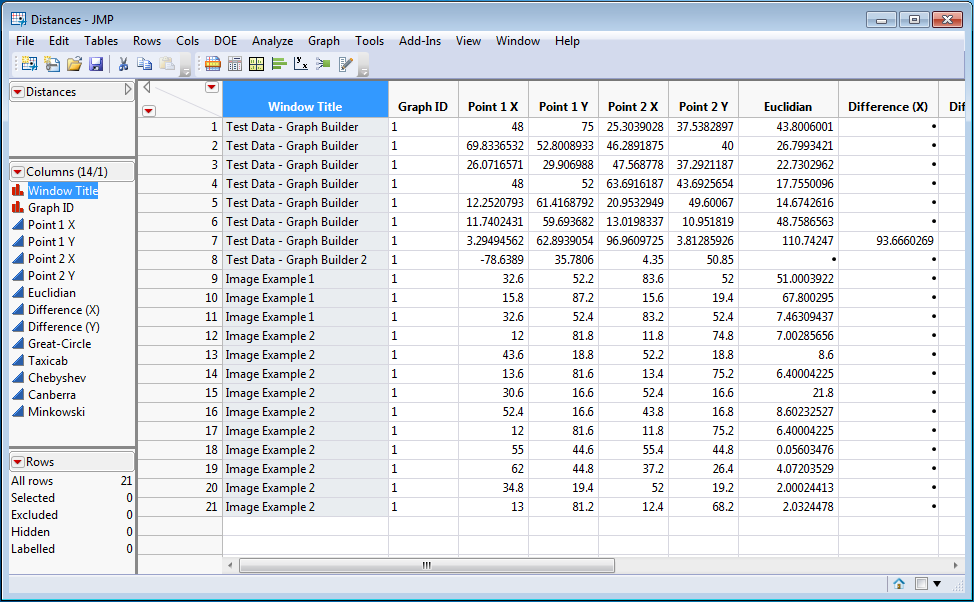Figure 5

The tool’s various options and features make it a powerful add-in for JMP.